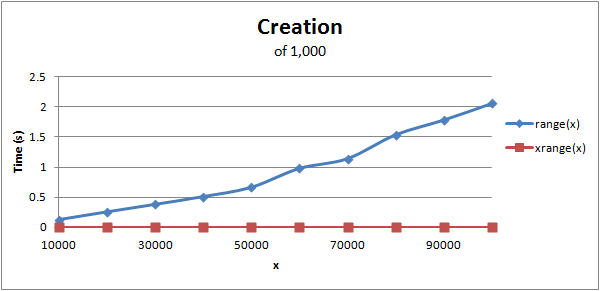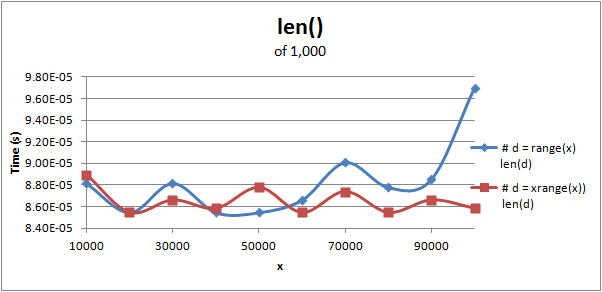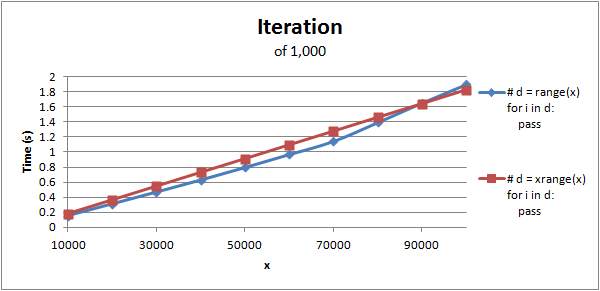## Tuesday, September 20, 2011

### Python: range() vs. xrange()

I recently discovered a really cool Python module called timeit. It let's you efficiently time blocks of Python code. So I decided to see the comparisons between range() and xrange(). I decided to look at a few aspects between them - the time to create them, compute the length (len()), and to iterate over them. As a note, each data point was achieved by running the block of code 1,000 times.

As many other websites state, there are cases when to use both. This is not a tutorial of what case is best for both, but rather a peek inside to help better understand them.We can clearly see that using range() increases linearly, algorithmically this is O(n). Using xrange() runs in constant time, O(1). Understanding the inner workings of the two reveals why. When using range, it goes ahead and computes all the numbers in the list and stores that array. This will cause it to use more memory since it has to store all the values. xrange is different in that it will compute the next number in the list when it is requested, not when it is created.Computing the length using the len() function can be approximated that it runs in linear time. Since the time to compute the length is fractions of a second, the consistency between testings varies (I ran this several times and nothing seemed to be very consistent). As a note, the creation of the range and xrange where not timed in the computing of the length.Most of the time these are used in a loop, but this tested just the basic iteration. Again the creation of the range and xrange were not timed. Since the iteration runs in linear time, we can assume that single element access to them run in a constant time, which is what is expected for both. Since normal iteration in code usually involves creating the range or xrange in the for statement, it is best to use xrange, especially for large values.

The script to create this data can be downloaded here. (Python 2.7 was used, but may work under different versions.)

As a side note, it is faster to run "a,b = b,a" than to run "t = a; a = b; b = t" to swap variables!

1.very nice explanation

2.This comment has been removed by a blog administrator.

3.In Python 3 we use the zip function which causes the iterations to loop at 32 million iterations per second on a 32 bit machine and 64 million on a 64 bit machine which is possible without len. That is why we improved it in 3. Len is discouraged in Python 3 also because the len used in a for loop in Python 3 causes the iterations to be double handled in the for loop.

And Python 2.7 is slower than the speed of zip unless you use iterzip. All Python 3 did was to connect iterzip/izip with len into zip for auto iterations without the need of a three to four module namespace pointers over-crossed as in Python 2.7, only one. And using Range and len outside of the Python 3 zip method is using Len and parts of izip with range/xrange which still exist in Py 3. Basically Python 3 changed nothing but improved 2.7's speed, pointer, len izip and range into one tightly bound package.

And len can still feed range before the loop begins then iterated through zip also but unnecessary. Zip is fast and directly runs in the C language loop. Python 2.7 has to store extra pointers in C to get the job done when Python 3's zip handles it all in one.

Misinformation is widespread on this topic but misusing the Python 3's for loop as a 2.7 loop isn't feasible. That is why we improved it. And it is faster than Python 2.7 using the zip. function with the correct methods in both. Many 2.7 users still cling to the old version 1.5 and 2.0 awkward iteration loop methods besides. Below is the correct tools for both Python 2.7 and 3's for loop iterations, which many developers never can fathom. So try using correct method tools in your test. You will love em.

Now on range Heres 2.7's tools:
__getitem__(...)
| x.__getitem__(y) <==> x[y]
|
| __iter__(...)
| x.__iter__() <==> iter(x)
|
| __len__(...)
| x.__len__() <==> len(x)
|
| __reduce__(...)
|
| __repr__(...)
| x.__repr__() <==> repr(x)
|
| __reversed__(...)
| Returns a reverse iterator

And here is Python 3's range tools:
__contains__(self, key, /)
| Return key in self.
|
| __eq__(self, value, /)
| Return self==value.
|
| __getitem__(self, key, /)
| Return self[key].
|
| __iter__(self, /)
| Implement iter(self).
|
| __len__(self, /)
| Return len(self).
|
| __ne__(self, value, /) #The 3's ability to allow leveling un-even pair iterations--
| Return self!=value.# --Allows the ability to add values to the shorter iterable.
|
| __repr__(self, /)
| Return repr(self).
|
| __reversed__(...)
| Return a reverse iterator.
|
| count(...) # "LEN UNNEEDED AND THIS METHOD IS WHY..."
| rangeobject.count(value) -> integer -- return number of occurrences of value
|
| index(...)
| rangeobject.index(value, [start, [stop]]) -> integer -- return index of value.
| Raise ValueError if the value is not present.

A bit behind is thy Holy Grail 2.7 users not the version lol. I use both but clearly Python 3.7 is my preferred version for iterations. And i test in 2.7 also. Keep it real bro ! Use both they are "One Python".